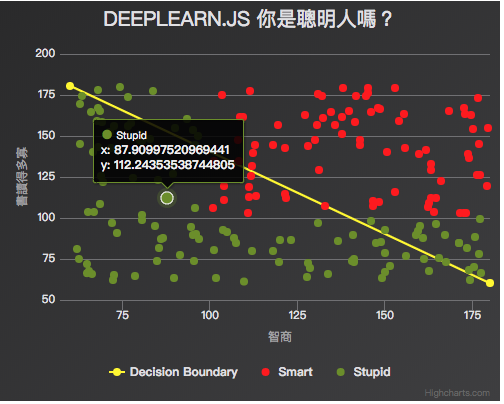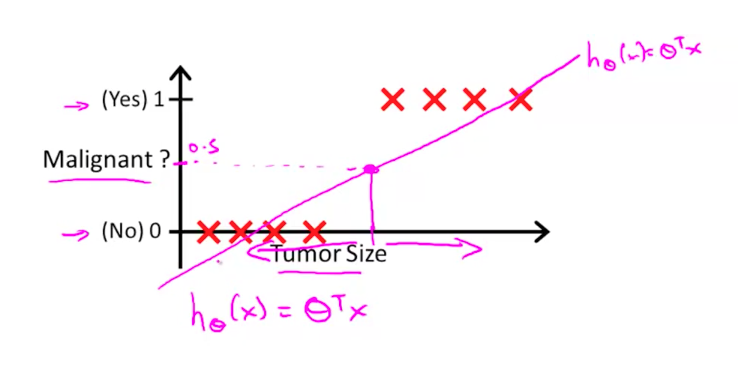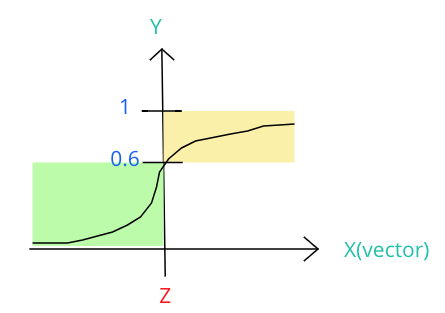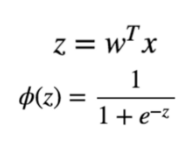# 前言## 出發總要有個方向，做實驗總要有個想像(圖片來源)## 大概了解 Logistic 後，那就來利用 deeplearn.js 找出 Decision Boundary 吧!

// 建立假資料，1 代表智商 100 分以上，0 代表智商 100 分以下
const data = []
for (let i=0; i<200; i++) {
let tmpX1 = Math.random() * 120 + 60;
let tmpX2 = Math.random() * 120 + 60;
data.push({
x: tmpX1,
y: tmpX2,
c: tmpX1 > 100 && tmpX2 > 100 ? 1: 0
});
}


/**
* deeplearn.js 運算
*/
const x_list = [];
const y_list = [];

for (let elem of data) {
x_list.push([elem.x, elem.y]);
y_list.push(elem.c);
}
const x_data = dl.tensor2d(x_list);
const y_data = dl.tensor2d(y_list);


• values (TypedArray|Array): tensor 的值。可以是 nested array 或 flat array 的結構。

• shape（number[]）:基本上就是該 tensor 的維度。若創建 tensor 時沒有指定維度，就會繼承傳入的 values 維度。也可以像這邊的範例一樣直接使用 tensor\${1|2|3|4}d 來創建

• dtype（float32'|'int32'|'bool）：值的型別，當然是 optional。

// 權重 W 與偏差 B
const W = dl.variable(dl.zeros([1, 2]));
const B = dl.variable(dl.zeros());


dl.variable(initialValue, trainable?, name?, dtype?) 用來創建 training 過程中需要的變數，也可透過參數指定該變數能否在 training 過程中被修改（trainable），預設是 true

dl.zeros([1, 2]) 來創建一個 Shape[1,2] 的填滿零值的 tensor 變數當權重 W，以及維度 1 的偏差變數 tensor B。

// 定義目標函數 與 loss function （最一般的 mean square）
// logistic regression 模型
const f = x => dl.sigmoid(W.matMul(x.transpose()));
// loss function（log loss）
const loss = (pred, label) => dl.mean(dl.neg(dl.sum(dl.mul(label, dl.log(pred)))));Z 就是我們要找的 boundary，就是權重與 input X 向量做矩陣乘法，所以這裡需要轉置矩陣 x.transpose()
dl.sigmoid() 就是 deeplearn.js 提供的 sigmoid 函數（如上圖第二行）

Z 帶入 dl.sigmoid() 後就獲得了目標函數 f。

// 梯度優化
const learningRate = 0.001
const optimizer = dl.train.sgd(learningRate)

// Training!
for (let i = 0; i < 1000; i++) {
optimizer.minimize(() => loss(f(x_data), y_data))
}


optimizer 可額外輸入兩個參數，分別控制 1. 是否回傳最後的 cost; 2. 限制只更新哪些變數。我們 for loop 1000 次後，利用 dataSync() 來將係數從 Tensor 讀出：

// 用 dataSync 取得 training 結果
const wPredict = W.dataSync();
const bPredict = B.dataSync();
console.log(wPredict, bPredict);


dataSync() 是 Synchronously 的，會 block Browser 的 UI thread，直到 data 被你讀出。另外還有個 Asynchronously 的 data() method，會回傳 promise，當讀取結束時再呼叫 resolves

// 計算切割線段
const data_line = [
[60, parseFloat((180 * wPredict + bPredict)/wPredict)],
[180, parseFloat((60 * wPredict + bPredict)/wPredict)]
];
console.log(data_line);


// 繪製圖形
const data_scatter1 = [];
const data_scatter2 = [];
for (let elem of data) {
if (elem.x > 100 && elem.y > 100) {
data_scatter1.push([elem.x, elem.y]);
} else {
data_scatter2.push([elem.x, elem.y]);
}
}

// Result
const options = {
title: {
text: 'deeplearn.js 你是聰明人嗎？'
},
xAxis: {
title: {
text: '智商'
},
min: 60,
max: 180
},
yAxis: {
title: {
text: '書讀得多寡'
},
min: 60,
max: 180
},
series: [
{
type: 'line',
name: 'Decision Boundary',
color: '#fff600',
data: data_line
},
{
type: 'scatter',
name: 'Smart',
marker: {
symbol: 'cross',
},
color: '#FF0000',
data: data_scatter1
},
{
type: 'scatter',
name: 'Stupid',
marker: {
symbol: 'cross',
},
color: '#6B8E23',
data: data_scatter2
}
]
};
// 图表初始化函数
const chart = Highcharts.chart('app', options);


## 最終成果

See the Pen DeeplearnJS-logistic-regression by Arvin (@arvin0731) on CodePen.

## 資料來源

@arvinh 前端攻城獅，熱愛數據分析和資訊視覺化